Some say that opposites attract. Others say that birds of a feather flock together. Which is it? Phillip N. Cohen, a professor of sociology at the University of Maryland, recently posted an interesting visualization that indicates that married couples who are college graduates tend to be birds of a feather. This article discusses Cohen's data and demonstrates some SAS techniques for assigning colors to a heat map, namely how to log-transform the response and how to bin the response variable by quantiles.

### Cohen's data and heat map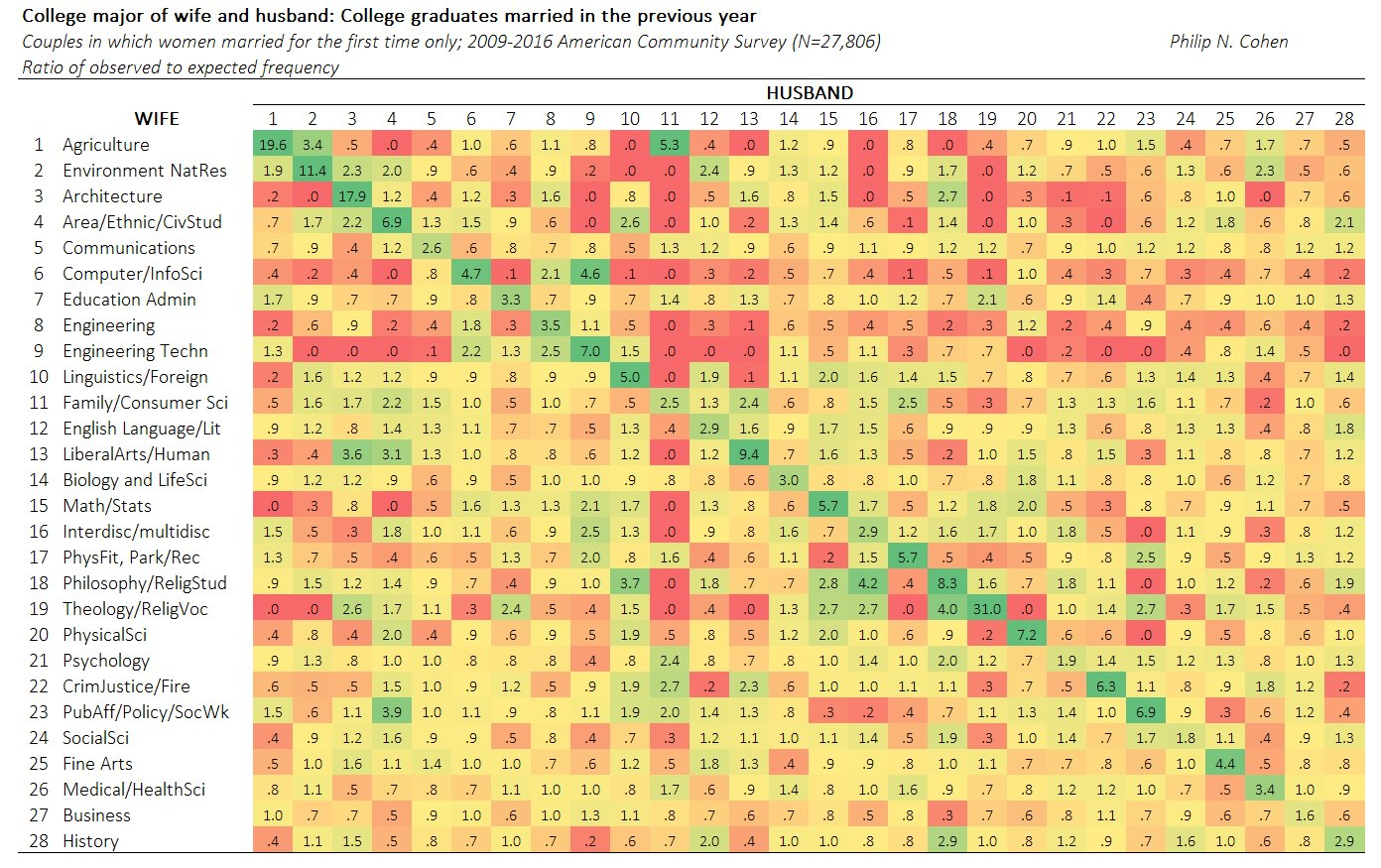Cohen cross-tabulated the college majors of 27,806 married couples from 2009–2016. The majors are classified into 28 disciplines such as Architecture, Business, Computer Science, Engineering, and so forth. The data are counts in a 28 x 28 table. If the (i,j)th cell in the table has the value C[i,j], then there are C[i,j] married couples in the data for which the wife had the i_th major and the husband has the j_th major.

Cohen used a heat map (shown to the right) to visualize associations among the wife's and husband's majors. This heat map shows the ratio of observed counts to expected counts, where the expected counts are computed by assuming the wife's major and the husband's major are independent. In this heat map, the woman's major is listed on the vertical axis; the man's major is on the horizontal axis. Green indicates more marriages than expected for that combination of majors, yellow indicates about as many marriages as expected, and red indicates fewer marriages than expected. The prevalence of green along the diagonal cells in the heat map indicates that many college-educated women marry a man who studied a closely related discipline. The couples are birds of a feather.

Cohen's heat map displays ratios. A ratio of 1 means that the observed number of married couples equals the expected number. For these data, the ratio ranges from 0 (no observed married couples) to 31. The highest ratio was observed for women and men who both majored in Theology and Religious Studies. The observed number of couples is 31 times more than expected under independence. (See the 19th element along the diagonal.)

### A heat map of relative differences

You might wonder why Cohen used the ratio instead of the difference between observed and expected. One reason to form a ratio is that it adjusts for the unequal popularity of majors. Business, engineering, and education are popular majors. Many fewer people major in religion and ethnic studies. If you don't account for the differences in popularity, you will see only the size of the majors, not the size of the differences.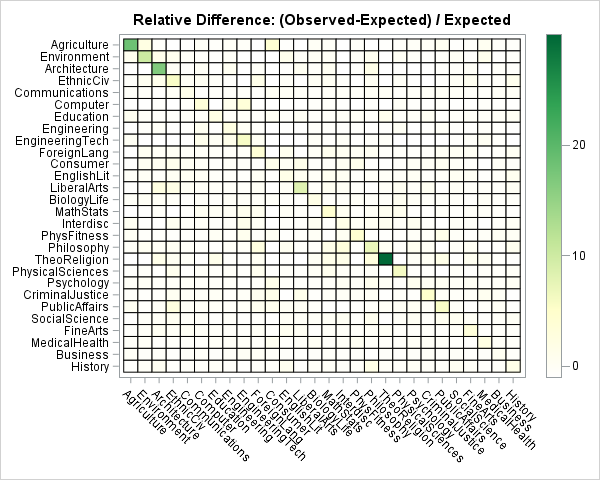A different way to view the data is to visualize the relative difference: (Observed – Expected) / Expected. The relative differences range from -1 (no observed couples) to 30 (for Theology majors). In percentage terms, this range is from -100% to 3000%. If you create a heat map of the relative difference and linearly associate colors to the relative differences, only the very largest differences are visible, as shown in the heat map to the right.

This heat map is clearly not useful, but it illustrates a very common problem that occurs when you use a linear scale to assign colors to heat maps and choropleth maps. Often the data vary by several orders of magnitude, which means that a linear scale is not going to reveal subtle features in the data. As shown in this example, you typically see a small number of cells that have large values and the remaining cell values are indistinguishable.

There are two common ways to handle this situation:

• If your audience is mathematically savvy, use a nonlinear transformation to map the values into a linear color ramp. A logarithmic transformation is the most common way to accomplish this.
• Otherwise, bin the data into a small number of discrete categories and use a discrete color ramp to assign colors to each of the binned values. For widely varying data, quantiles are often an effective binning strategy.

### Log transformation of counts

A logarithmic transform (base 10) is often the simplest way to visualize data that span several orders of magnitudes. When the data contain both positive and negative values (such as the relative differences), you can use the log-modulus transform to transform the data. The log-modulus transform is the transformation x → sign(x) * log10(|x| + 1). It preserves the sign of the data while logarithmically scaling it. The following heat map shows the marriage data where the colors are proportional to the log-modulus transform of the relative difference between the observed and expected values.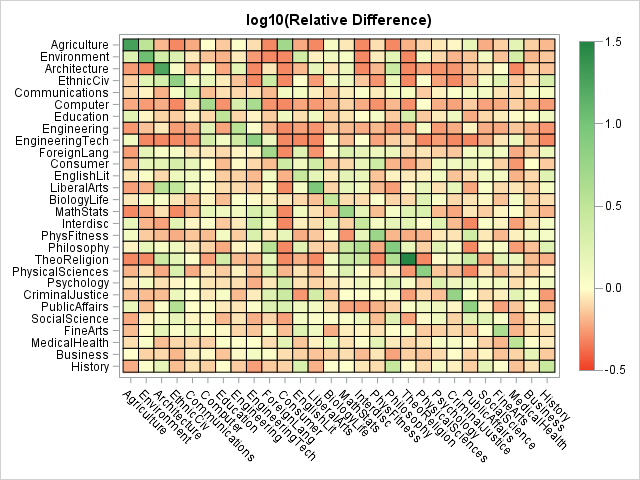As in Cohen's heat map of the ratios, mapping a continuous color ramp onto the log-transformed values divides the married couples into three visual categories: The reddish-orange cells indicate fewer marriages than expected, the cream-colored cells indicate about as many marriages as expected, and the light green and dark green cells indicate many more marriages than expected. The drawback of the log transformation is that the scale of the gradient color ramp is not easy to understand. I like to add tooltips to the HTML version of the heat map so that the user can hover the mouse pointer over a cell to see the underlying values.

### Analysis of the marriage data

What can we learn about homophily (the tendency for similar individuals to associate) from studying this heat map? Here are a few observations. Feel free to add your own ideas in the comments:

• The strongest homophily is along the diagonal elements. In particular, Theology/Religion majors are highly likely to marry each other, as are agriculture, environment, and architecture majors.
• Female engineers, computer scientists, and mathematicians tend to marry like-minded men. Notice the large number of red cells in those rows for the non-mathematical disciplines.
• Women in medical/health field or business are eclectic. Those rows do not have many dark-shaded cells, which indicates a general willingness to associate with men inside and outside of their subject area.

### Discrete heat map by quantiles

It is easiest to interpret a heat map when the colors correspond to the actual counts or simple statistics such as the relative difference. The simplest way to assign colors to the cells in the heat map is to bin the data. This results in a discrete set of colors rather than the continuous color ramp in the previous example. You can bin the data by using five to seven quantiles. Alternatively, you can use domain-specific or clinical ranges. (For example, you could use clinical "cut points" to bin blood pressure data into "normal," "elevated," and "hypertensive" categories. In SAS, you can use PROC FORMAT to create a customized format for the response variable and color according to the formatted values.

The following heat map uses cut points to bin the data according to the relative difference between observed and expected values. As before, red and orange cells indicate observed differences that are negative. The cream and light green cells indicate relative differences that are close to zero. The darker greens indicate observed differences that are a substantial fraction of the expected values. The darkest green indicates cells for which the difference is more than 100% greater than the expected value.

When you use a discrete color ramp, all values within a specified interval are mapped to the same color. In this heat map, most cells on the diagonal have the same color; you cannot use the heat map to determine which cell has the largest relative difference.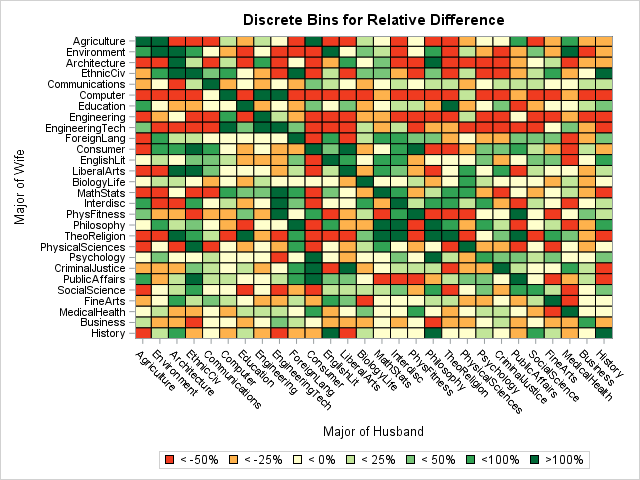### Summary

There is much more to say about these data and about ways to use heat maps to discover features in the data. However, I'll stop here. The main ideas of this article are:

• The data are interesting. In general, the married couples in this study tended to have similar college majors. The strength of that relationship varied according to the discipline and also by gender (the heat map is not symmetric). For more about the data, see Professor Cohen's web page for this study.
• When the response variable varies over several orders of magnitude, a linear mapping of colors to values is not adequate. One way to accommodate widely varying data is to log-transform the response. If the response has both positive and negative values, you can use the log-modulus transform.
• For a less sophisticated audience, you can assign colors by binning the data and using a discrete color ramp.

The post Assign colors in heat maps: A study of married couples and college majors appeared first on The DO Loop.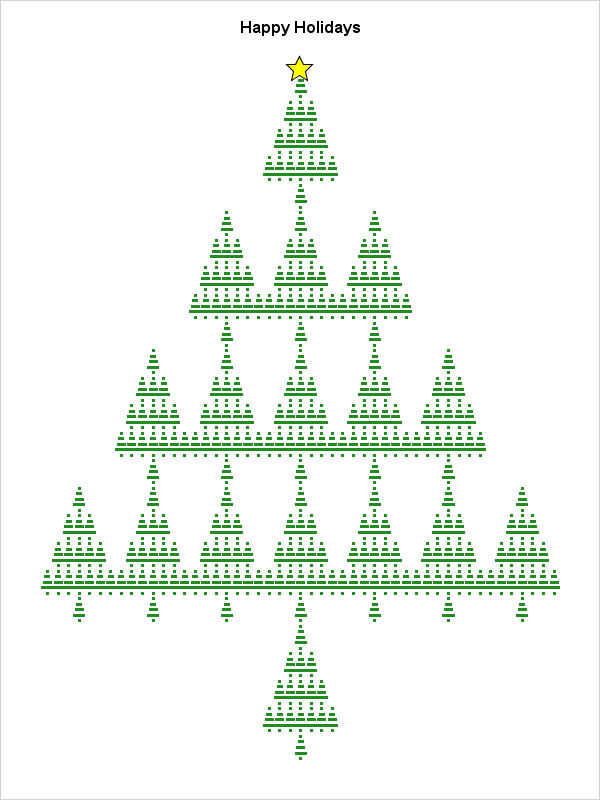Happy holidays to all my readers! My greeting-card to you is an image of a self-similar Christmas tree. The image (click to enlarge) was created in SAS by using two features that I blog about regularly: matrix computations and ODS statistical graphics.

### Self-similarity in Kronecker products

I have previously shown that the repeated Kronecker product of a binary matrix leads to self-similar structures.

Specifically, if M is a binary matrix then the Kronecker product M@M is a block matrix in which each 1 in the original matrix is replaced with a copy of M and each 0 is replaced by a zero block. (Here '@' is the Kronecker product (or direct product) operator, which is implemented in SAS/IML software.) If you repeat this process three or more times and create a heat map of the product matrix, the self-similar structure is apparent.

For example, the following 5 x 7 binary matrix has a 1 in positions that approximate the shape of a Christmas tree. The direct product M@M@M is a matrix that has 53 = 125 rows and 73 = 343 columns. If you use the HEATMAPDISC subroutine in SAS/IML to create a heat map of the direct product, you obtain the following image in which a green cell represents a 1:

```proc iml; M = {0 0 0 1 0 0 0, 0 0 1 1 1 0 0, 0 1 1 1 1 1 0, 1 1 1 1 1 1 1, 0 0 0 1 0 0 0}; Tree = M @ M @ M; /* M is 5 x 7, so Tree is 125 x 343 */   ods graphics / width=300px height=450px ANTIALIASMAX=50000; call heatmapdisc(Tree) title="Happy Holidays to All my Readers!" colorramp={White Green} displayoutlines=0 ShowLegend=0;```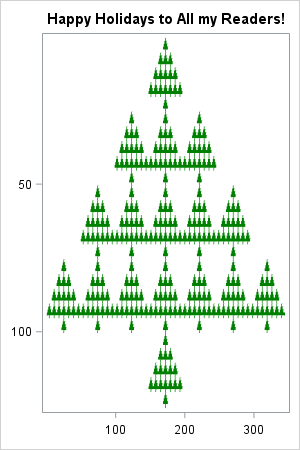That's not a bad result for three SAS/IML statements! You can see that the "tree" has a self-similar structure: the large tree is composed of 17 smaller trees, each of which is composed of 17 mini trees.

### Adding a star

My readers are worth extra effort, so I want to put a star on the top of the tree. One way to do this is to use a scatter plot and plot a special observation by using a star symbol. To plot the "tree" by using a scatter plot, it is necessary to "unpack" the 125 x 343 matrix into a list of (x, y) values (this is a wide-to-long conversion of the matrix). You can use the NDX2SUB function to extract the row and column of each 1 in the matrix, as follows:

```/* extract (row, column) pairs for matrix elements that are '1' */ idx = loc(Tree = 1); /* indices for the 1s */ s = ndx2sub(dimension(Tree), idx); /* convert indices to subscripts */ create Tree from s[c={"RNames" "CNames"}]; append from s; close;   call symputx("XPos", range(s[,2])/2); /* midrange = horiz position of star */ quit;```

The previous statements create a SAS data set called TREE that contains the (x, y) positions of the 1s in the direct product matrix. The statements also saved the midrange value to a macro variable. The following SAS procedures create the location of the star, concatenate the two data sets, and create a scatter plot of the result, which is shown at the top of this article.

```data star; x = &XPos; y = 0; run;   data Tree2; set Tree star; run;   ods graphics / width=600px height=800px ANTIALIASMAX=50000; title "Happy Holidays"; proc sgplot data=Tree2 noborder noautolegend; scatter x=CNames y=RNames / markerattrs=(color=ForestGreen symbol=SquareFilled size=3); scatter x=x y=y / markerattrs=(symbol=StarFilled size=20) /* yellow star */ filledoutlinedmarkers markerfillattrs=(color=yellow); yaxis reverse display=none; xaxis display=none; run;```

### Previous SAS-created Christmas cards

All year long I blog about how to use SAS for serious pursuits like statistical modeling, data analysis, optimization, and simulation. Consequently, I enjoy my occasional forays into a fun and frivolous topic such as how to create a greeting card with SAS. If you like seeing geeky SAS-generated images, here is a list of my efforts from previous years:

Wherever you are and whatever holiday you celebrate, may you enjoy peace and happiness this season and in the New Year.

The post A self-similar Christmas tree appeared first on The DO Loop.Last week I discussed how to create spaghetti plots in SAS. A spaghetti plot is a type of line plot that contains many lines. Spaghetti plots are used in longitudinal studies to show trends among individual subjects, which can be patients, hospitals, companies, states, or countries. I showed ways to ease overplotting in spaghetti plots, but ultimately the plots live up to their names: When you display many individual curves the plot becomes a tangled heap of noodles that is hard to digest.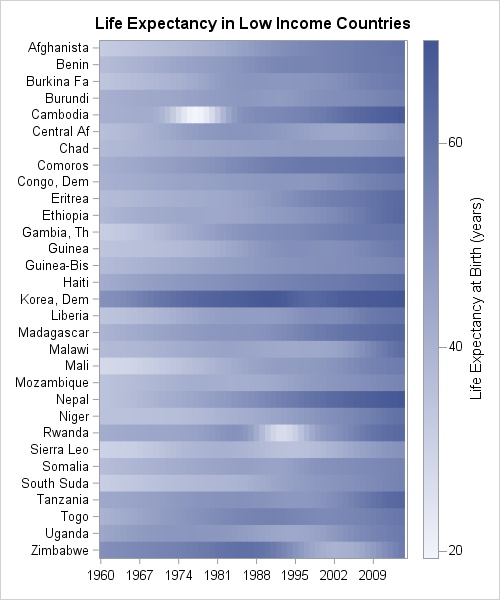An alternative is to use a heat map instead of a line plot, as shown to the left. (This graph is created later in this article.) Each row of the heat map represents a subject. Each column represents a time point. Heat maps are useful when a response variable is recorded for every individual at the same set of uniformly spaced time points, such as daily, monthly, or yearly.

Swihart et al. (2010) proposed the name "lasagna plot" to denote a heat map that visualizes longitudinal data. Whereas the spaghetti plot allows "noodles" (the individual curves) to cross, a lasagna plot layers the noodles horizontally, one on top of another. A related visualization is a calendar chart (Mintz, Fitz-Simons, and Wayland (1997); Allison and Zdeb (2005)), which also uses colored tiles to convey information about a response variable as a function of time.

This article shows how to create lasagna plots in SAS. To create a lasagna plot in SAS you can:

Create a lasagna plot in #SAS, because sometimes spaghetti plots don't satisfy. #DataViz
Click To Tweet

### Create a basic lasagna plot in SAS

In a previous article I showed how to download the World Bank data for the average life expectancy in more than 200 countries during the years 1960–2014. After downloading the data, the data are transformed from "wide form" into "long form." The following call to PROC SGPLOT creates a spaghetti plot of the "Low Income" countries.

```ods graphics / imagemap=ON; /* enable data tips */ title "Life Expectancy at Birth"; title2 "Low-Income Countries"; proc sgplot data=LE; /* create conventional spaghetti plot */ where income=5; /* extract the "low income" companies */ format Country_Name \$10.; /* truncate country names */ series x=Year y=Expected / group=Country_name break curvelabel lineattrs=(pattern=solid) tip=(Country_Name Region Year Expected); run;```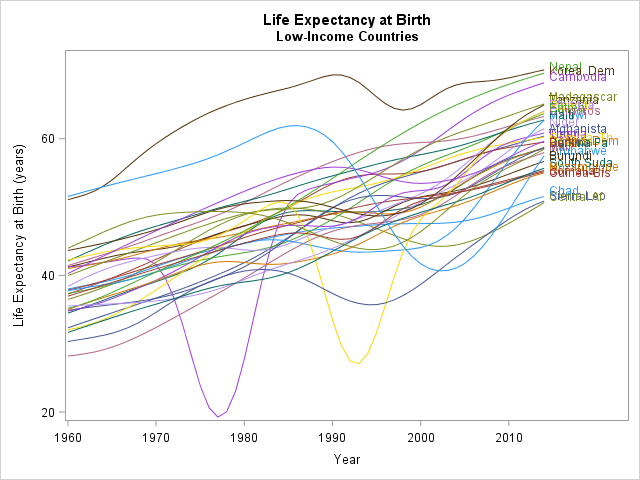This spaghetti plot is not very enlightening. There are 31 curves in the graph, although discovering that number from the graph is not easy. The labels that identify the curves overlap and are impossible to read. You can see some trends, such as the fact that life expectancy has, on average, increased for these countries. You can also see some interesting features. Cambodia (a reddish color) experienced a dip in the 1970s. Rwanda (purple) and Sierra Leone (gold) experienced dips in the 1990s. Zimbabwe (light blue) experienced a big decline in the 2000s.

A lasagna plot visualizes these data more effectively. The following statements use the HEATMAP statement in PROC SGPLOT, which requires SAS 9.40M3:

```title "Life Expectancy in Low Income Countries"; /* 1. Unsorted list of low-income countries */ ods graphics/ width=500px height=600px discretemax=10000; proc sgplot data=LE; where Income=5; /* extract the "low income" companies */ format Country_Name \$10.; /* truncate country names */ heatmap x=Year y=Country_Name/ colorresponse=Expected discretex colormodel=TwoColorRamp; yaxis display=(nolabel) labelattrs=(size=6pt) reverse; xaxis display=(nolabel) labelattrs=(size=8pt) fitpolicy=thin; run;```

The graph is shown at the top of this article. Each row is a country; each column is a year. The default two-color color ramp encodes the value of the response variable, which is the average life expectancy. There are 31 x 55 = 1705 tiles, so the image displays a lot of information without any overplotting. You can use the COLORMODEL= option on the HEATMAP statement to specify a custom color ramp.

Many rows have a light shade on the left and a darker shade to the right, which confirms the general upward trend of the response variable. Countries that experienced a period of decline in life expectancy (Cambodia, Rwanda, and Zimbabwe) have a region of white or light blue in the middle of the row. In some countries the life expectancy has been consistently low (Chad and Guinea-Bissau); in others it has been consistently high (Dem. People's Republic of Korea).

The lasagna plot is not perfect. Because the graph avoids overplotting, you need a lot of space to display the rows. This lasagna plot uses 600 vertical pixels to display 31 countries. That's about 16 pixels for each row after you subtract the space above and below the heat map. If you use a smaller font, you can reduce that to 10 or 12 pixels per row. However, even at 12 pixels per row you would need about 2500 pixels in the vertical direction to display all 207 countries in the data set. In contrast, the spaghetti plot displays an arbitrary number of (possibly undecipherable) lines in a smaller area.

### Sorting rows of a lasagna plot

Alphabetical ordering is often not the most informative way to display the levels of a categorical variable. You can sort the countries in various ways: by the mean life expectancy, by the average rate of increase (slope), by geographical region, and so forth.

To demonstrate sorting, the following program uses the HEATMAPCONT subroutine in the SAS/IML language. The following statements read in data for 51 lower-middle income countries into a SAS/IML matrix. The statements read the original "wide" data whereas PROC SGPLOT required "long" data. Use the PALETTE function to create a custom color ramp for the heat map.

```ods graphics / width=500px height=650px; proc iml; varName = "Y1960":"Y2014"; use LL2 where (Income=4); /* read "lower-middle income" countries */ read all var varName into X[rowname=Country_Name]; /* X = "wide" data matrix */ close LL2;   Names = putc(Country_Name, "\$15."); /* truncate names */ palette = "CXFFFFFF" || palette("YLORRD", 4); /* palette from colorbrewer.org */   /* 2. Order rows by average life expectancy from 1960-2014 */ mean = X[,:]; /* compute mean for each row */ call sortndx(idx, mean, 1, 1); /* sort by first column, descending */ Sort1 = X[idx,]; /* use sorted data */ Names1 = Names[idx,]; /* and sorted names */ call heatmapcont(Sort1) xvalues=1960:2014 yvalues=Names1 displayoutlines=0 colorramp=palette title="Life Expectancy Sorted by Average";```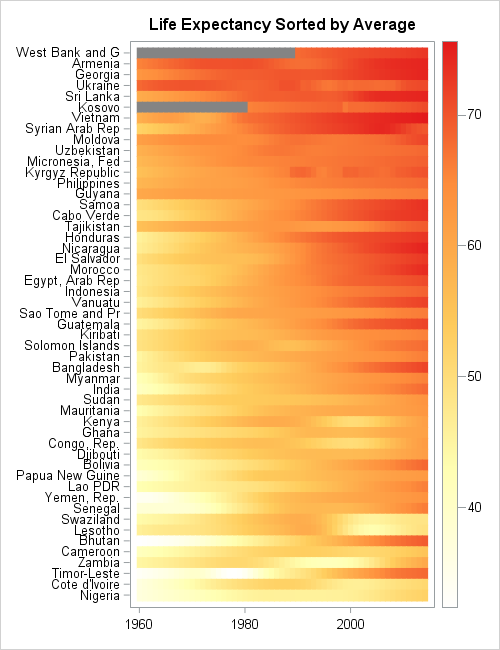The lasagna plot show the life expectancy for 51 countries. The countries are sorted according to mean life expectancy over the 55 years in the data.

The sorted visualization is superior to the unsorted version. You can easily pick out the countries that have the top life expectancy, such as former Soviet Union countries. you can easily see that the countries at the bottom of the list are mainly in Western and Southern Africa.

The countries that experienced dips in life expectancy contain a patch of white or pale yellow in the middle of the row. Zambia, Kenya, and Lesotho stand out. Countries that have dramatically improved their life expectancy are also evident. For example, the rows for Bhutan and Timor-Leste are white or yellow on the left and dark orange on the right, which indicates that life expectancy has greatly improved in the past 55 years for these countries.

In this heat map, missing values are assigned a gray color. Only two countries (West Bank and Gaza, Kosovo) have missing values for life expectancy.

### Other ways to sort lasagna plots

Swihart et al (2010) discuss other sorting techniques. One alternate display is to sort each column independently. This is similar to displaying a sequence of box plots, one for each year, because it shows the distribution of the response variable for each year, aggregated over all countries. This display is accomplished by using the following SAS/IML statements to sort each column in the data matrix:

```/* 3. Order each year to see distribution of life expectancy for each year */ Sort2 = X; /* copy original data */ do i = 1 to ncol(X); v = X[,i]; /* extract i_th column */ call sort(v, 1, 1); /* sort i_th column descending */ Sort2[,i] = v; /* put sorted column into matrix */ end; call heatmapcont(Sort2) xvalues=1960:2014 displayoutlines=0 colorramp=palette title="Life Expectancy Sorted for each Year";```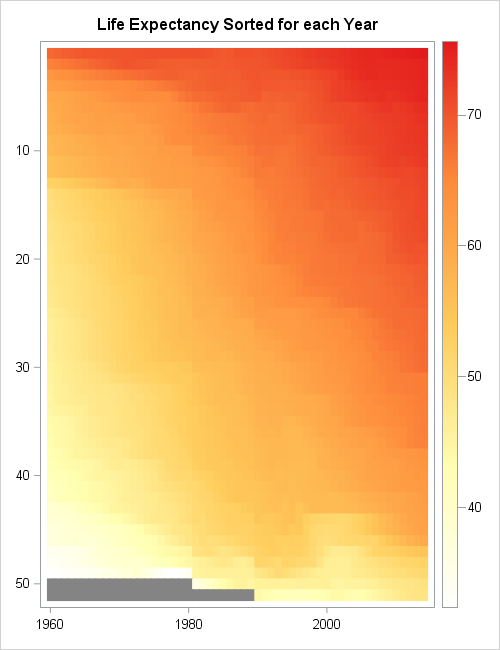In this graph, each tile still represents a country, but you don't know which country. The purpose of the graph is to show how the distribution of the response variable has changed over time. You can see that in 1960 most countries had a life expectancy of 55 years or less, as shown by the vertical strip of mostly yellow tiles for 1960. Only one country in 1960 had an average life expectancy that approached 70 years (red).

Decades later, the vertical strips contain fewer yellow tiles and more orange and red tiles. The strip for 1990 contains mainly orange or dark orange tiles, which indicates that most countries have an average life expectancy of 55 years or greater. For 2014, more than half of the vertical strip is dark orange or red, which indicates that most countries have an average life expectancy of 65 years or greater.

### Summary

A "lasagna plot" is a heat map in which each row represents a subject such as a country, a patient, or a company. The columns usually represent time, and the colors for each tile indicate the value of a response variable. The lasagna plot is useful when the response is measured for all subjects at the same set of uniformly spaced time points.

In contrast to spaghetti plots, there is no overplotting in a lasagna plot. However, each subject requires some minimal number of vertical pixels (usually 10 or more) so lasagna plots are not suitable for visualizing thousands of subjects. Lasagna plots are most effective when you sort the subjects by some characteristic, such as the mean response value.

Much more can be said about lasagna plots. I recommend the paper "A Method for Visualizing Multivariate Time Series Data," (Peng, JSS, 2008), which discusses techniques for standardizing the data, smoothing the data, and discretizing the response variable. Peng's article includes panel displays, which can be created in SAS by using the GTL and PROC SGRENDER.

The post Lasagna plots in SAS: When spaghetti plots don't suffice appeared first on The DO Loop.When SAS 9.4m3 was released last month (including SAS/STAT and SAS/IML 14.1), I was happy to see that a HEATMAP statement had been added to the SGPLOT procedure. Although heat maps in the SAS/IML language have been available for several releases, you previously had to use the Graph Template Language (GTL) to create a customized heat map in Base SAS.

To illustrate using the HEATMAP statement, consider the following counts for patients in a medical study, classified by ordinal variables that represent the patients' weight and smoking status. The data and the techniques used to create the table are discussed in the article "How to order categories in a two-way table with PROC FREQ." You can download the complete SAS program that is used to create the table and graphs in this analysis.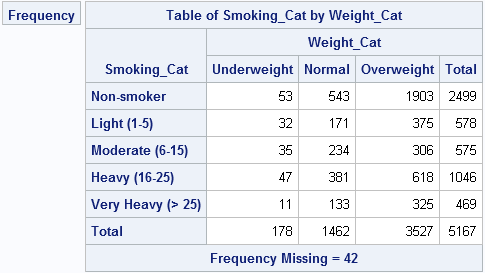The categories and counts are contained in a data set called FreqOut, which was created by the FREQ procedure. The data set is arranged in "list form" (or "long form") as follows: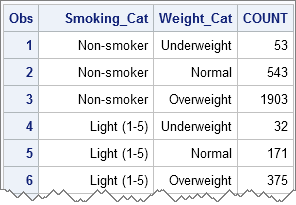### A basic heat map of a frequency table

The following statements create a basic heat map of the frequencies for the 15 cells of the table:

```proc sgplot data=FreqOut; heatmap x=Weight_Cat y=Smoking_Cat / freq=Count discretex discretey colormodel=TwoColorRamp outline; run;```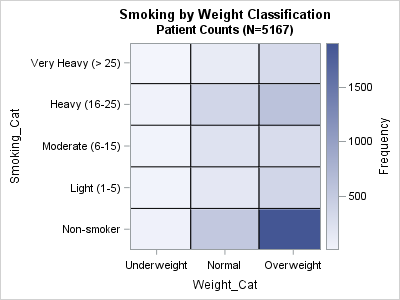On the HEATMAP statement, the DISCRETEX and DISCRETEY options are used because the underlying variables are numeric; a user-defined format is used to render the numbers as "Underweight," "Normal," "Overweight," and so forth. A two-color gradient ramp is good for showing counts. The default color ramp has three colors (blue, white, and red), which is good for visualizing deviations from a reference value.

The heat map makes it visually clear that most patients in the study are overweight non-smokers. Other categories with many patients include the overweight heavy-smokers, the normal-weight non-smokers, and the normal-weight heavy-smokers. Underweight patients (regardless of smoking status) are relatively rare.

### Overlaying text on a heat map

Although this same heat map can be created in SAS/IML by using the HEATMAPCONT subroutine, using PROC SGPLOT makes it easy to overlay other plot types and change properties of the graph. For example, you might want to overlay the counts of each cell to create a color-coded table. You can easily add the TEXT statement to the previous SGPLOT statements. If you add the counts, you don't need to include the gradient color ramp. Furthermore, to make the heat map look more like the table, you can add the REVERSE option to the YAXIS statement. Lastly, the category labels make it clear that the variables are related to smoking and weight, so you can suppress the variable names. The following statements implement these revisions and create a new heat map:

```proc sgplot data=FreqOut noautolegend; heatmap x=Weight_Cat y=Smoking_Cat / freq=Count discretex discretey colormodel=TwoColorRamp outline; text x=Weight_Cat y=Smoking_Cat text=Count / textattrs=(size=16pt); yaxis display=(nolabel) reverse; xaxis display=(nolabel); run;```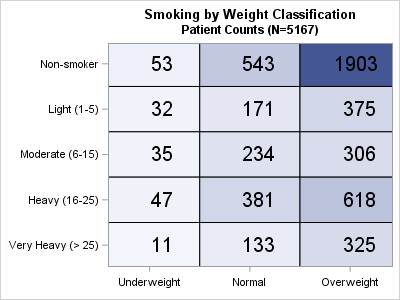I'm excited by the HEATMAP statement in PROC SGPLOT. I think it is a great addition to one of my favorite SAS procedures.

tags: 14.1, Heat maps

The post Create heat maps with PROC SGPLOT appeared first on The DO Loop.I recently posted an article about self-similar structures that arise in Pascal's triangle. Did you know that the Kronecker product (or direct product) can be used to create matrices that have self-similar structure?

The basic idea is to start with a 0/1 matrix and compute a sequence of direct products of the matrix with itself. If M is a matrix that contains only zeros and ones, then M@M is a block matrix where a block of zeros occurs where M has a 0, and a copy of M appears where M has a 1, as shown by the following SAS/IML program:

```proc iml; M = {0 1 0, 1 1 1, 0 1 0}; M2 = M @ M; print M2;```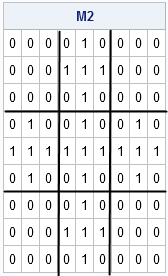In this example, the original matrix, M, is 3 x 3. The matrix M@M is a 3 x 3 block matrix, where each block is either the zero matrix or a copy of M. If you apply the Kronecker product again and form the matrix M@M@M, you will get a 9 x 9 block matrix, where each block is either the zero matrix or a copy of M.

You can continue this process. The k-fold product of M with itself is a 3k x 3k matrix. Arthur Charpentier provides a nice animation of this iterative process on his Freakonometrics blog, which is where I first read about using the Kronecker product of a 0/1 matrix to create a self-similar structure. If you like to read articles about mathematical aspects of probability and statistics, I highly recommend Charpentier's well-written and always interesting blog.

Printing a 0/1 matrix is not an effective visualization. Instead, I will create a two-color heat map by using the HEATMAPDISC subroutine in SAS/IML 13.1. Because I want to create several heat maps, I wrote the following SAS/IML module that composes a matrix with itself k times and draws a two-color heat map of the result. The following heat map shows the structure of the four-fold Kronecker product M@M@M@M:

```start KroneckerPlot(M, Iters); N = 1; do i = 1 to Iters; N = N @ M; end; call heatmapdisc(N) title="Iteration: "+strip(char(Iters)) colorramp={White Blue} displayoutlines=0 ShowLegend=0; finish;   ods graphics / width=800px height=800px; run KroneckerPlot(M, 4);```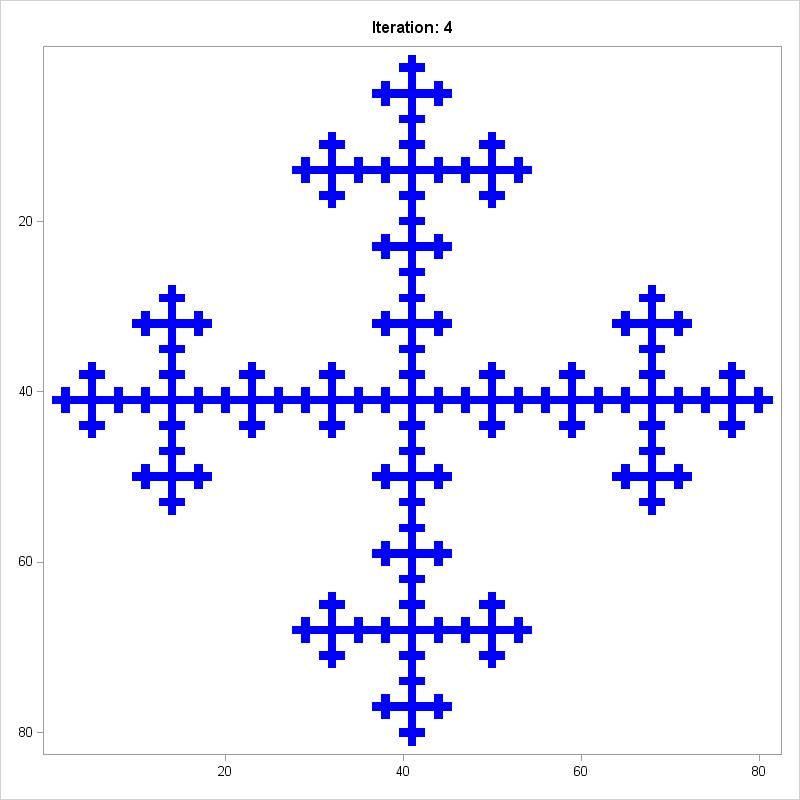Let's say it all together: "Ooooooh!" "Ahhhhh!"

The structures that you can create depend on the arrangement of zeros and ones in the original matrix. Notice that these product matrices get big fast: the k-fold Kronecker product of an n x n matrix is an nk x nk matrix. You might run out of memory if you try to visualize a k-fold product when k or n is greater than 5. The next statements start with a 4 x 4 matrix and display a heat map for the five-fold product, which is a 1024 x 1024 matrix:

```M = {1 1 1 1, 1 1 0 0, 1 0 1 0, 1 0 0 1}; run KroneckerPlot(M, 5); /* 5 iterations of 4x4 matrix ==> 1024 x 1024 matrix */```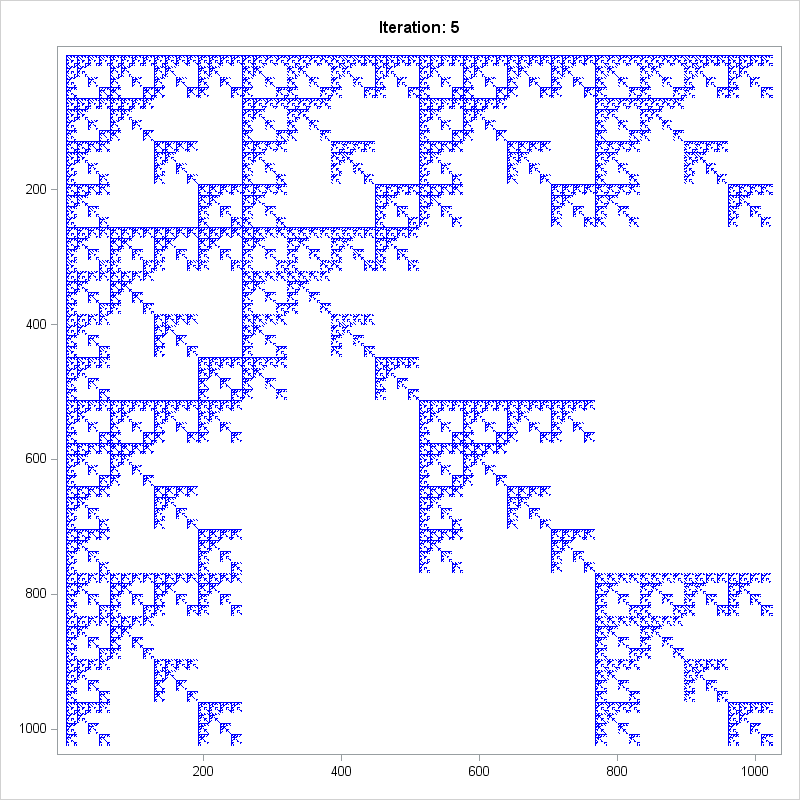I call this image "Landing at LaGuardia."

Obviously you can create other structures. You can even generate random structures by using the Bernoulli distribution to create a random 0/1 matrix. I leave you with a few additional statements that create self-similar images. Feel free to create your own matrices. Leave a comment and tell me your favorite matrix for creating a self-similar structure.

```ods graphics / width=500px height=500px; M = {1 1 1 1, 1 0 0 1, 1 0 0 1, 1 1 1 1}; run KroneckerPlot(M, 4);   /* Make random fractal plots! */ call randseed(54321); do i = 1 to 5; M = randfun({4 4}, "Bernoulli", 0.75); run KroneckerPlot(M, 3); end;```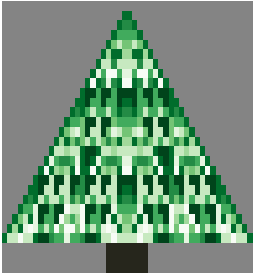O Christmas tree,
O Christmas tree,
One year a fractal made thee!
O Christmas tree,
O Christmas tree,
A heat map can display thee!

From Pascal's matrix we define!
Reflect across, divide by nine.
O Christmas tree,
O Christmas tree,
Self-similar and so divine!

Eventually I will run out of cute ways to use SAS software to create and visualize a Christmas-themed image. But not this year!

My recent article about how to create Pascal's matrix in SAS included a lower triangular matrix that exhibits self-similar patterns. If you take the Pascal matrix modulo 9 and reflect it across the Y axis, you get a triangular array that looks a little bit like a Christmas tree. You can add a "trunk" to the bottom of the tree to improve the resemblance. As shown in my previous post, you can use a heat map to visualize the matrix. In the following SAS/IML program, I use a green palette of colors to visualize the Pascal triangle values modulo 9. The resulting heat map is shown at the top of this article.

```proc iml; start PascalRule(n); m = j(n,n,0); /* initialize with zeros */ m[,1] = 1; /* set first column to 1 */ j = 2:n; /* elements to compute */ do k = 2 to n; /* for kth row, add adjacent elements from previous row */ m[k,j] = m[k-1,j-1] + m[k-1,j]; end; return(m); finish;   /* reflect Pascal's triangle to create left-right symmetry and add a tree trunk to create a Christmas tree image */ start PascalTree(n); m = PascalRule(n); T = m[,n:2] || m[,2:n]; /* reflect; omit column of 1s */ T[ loc(T=0) ] = .; /* replace zeros with missing values */ trunk = j(3,ncol(T),.); /* add a "tree trunk" with value -1 */ midpt = ncol(T)/2; /* note that ncol(T) is even */ halfwidth = ceil(n/10); trunk[,(midpt-halfwidth):(midpt+halfwidth+1)]= -1; return( T // trunk ); finish;   m = PascalTree(25); k = 9; tree = mod(m,k);   ods graphics / width=300px height=380px; title = "Happy Holidays to All SAS Users!"; ramp = palette("greens", k); ramp = "CX26261C" || ramp[,k:1]; /* brown (for trunk) and green palette */ call heatmapdisc(tree) colorramp=ramp displayoutlines=0 title=title;```

It is remarkable that the Pascal matrix has so many amazing mathematical properties. Now you can add "makes a reasonable facsimile of a Christmas tree" to the list!

Happy holidays and a wonderful New Year to all of my readers. You are the reason that I write this blog.

tags: Heat maps, Just for FunPascal's triangle is the name given to the triangular array of binomial coefficients. The nth row is the set of coefficients in the expansion of the binomial expression (1 + x)n. Complicated stuff, right?

Well, yes and no. Pascal's triangle is known to many school children who have never heard of polynomials or coefficients because there is a fun way to construct it by using simple addition. You start by writing down the number 1. The second line is the sum of the first line and the result of shifting the first line one column to the right. This process continues: to form a new row of the triangle, add the previous row to a right-shifted copy of the previous row. To make the bookkeeping work out, you pretend that missing items are zero, as shown by the ghostly images of zeros in the image at the top of this article.

In math terms, you can find the kth element of the nth row, by adding the (k–1)th and the kth elements of the previous row. This geometric construction is a result of the following recursive relationship between binomial coefficients: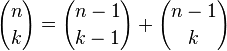Recently several participants on the SAS/IML Support Community posted programs that created Pascal's triangle by using the COMB function in SAS software. This article shows how to create Pascal's triangle and how self-similar (fractal) patterns arise when you visualize the triangle in certain ways.

### Creating an array of binomial coefficients

The simplest way to create Pascal's matrix is to use the COMB function to generate the values of the binomial coefficients. You can start with a matrix that contains all zeros. The first k elements of the kth row are filled with binomial coefficients. You can do this in the SAS DATA step, or in the SAS/IML language as follows:

```proc iml; start PascalTriangle(n); m = j(n,n,0); /* matrix with all zeros */ do k = 1 to n; /* for the kth row... */ m[k,1:k] = comb(k-1, 0:k-1); /* fill nonzero elements */ end; return(m); finish;   T10 = PascalTriangle(10); print T10[F=3. L="Pascal's Triangle, Level 10" r=("n=0":"n=9")];```

The resulting matrix is similar to the image at the top of this post, with the upper triangular elements equal to zero. Notice that the SAS/IML language enables you to pass in a vector argument to a Base SAS function. In this case, a vector (0:k-1) is passed to the COMB function and the resulting row vector overwrites some of the zeros in the matrix m.

### Using the previous row to create the next row of Pascal's matrix

You can also create Pascal's matrix by using the "schoolchild method" of adding adjacent elements from the previous row to create the new row. The construction is similar to the way that you can construct cellular automata. The following SAS/IML module constructs Pascal's triangle by using addition; no need to call the COMB function!

```start PascalRule(n); m = j(n,n,0); /* initialize with zeros */ m[,1] = 1; /* set first column to 1 */ j = 2:n; /* elements to compute */ do k = 2 to n; /* for kth row, add adjacent elements from previous row */ m[k,j] = m[k-1,j-1] + m[k-1,j]; end; return(m); finish;   T10 = PascalRule(10); print T10[F=3. L="Pascal's Triangle, Level 10" r=("n=0":"n=9")];```

### Self-similar structures in Pascal's triangle

At first glance, the numbers in Pascal triangle have a simple structure. The edges of the triangle are all 1. The interior values increase geometrically, reaching their maximum values in the middle of the final row. However, if you label each value according to whether it is odd or even, a surprising pattern reveals itself!

The following SAS/IML program creates a Pascal matrix with 56 rows. The upper-triangular elements are set to missing values. The program then creates a discrete heat map that shows the parity (even or odd) of the remaining elements. Even numbers are displayed as white squares; odd numbers are displayed as black squares.
```ods graphics / width=400px height=380px; m = PascalRule(56); m[ loc(col(m)>row(m)) ] = .; /* replace zeros with missing values */ mod2 = mod(m,2); call heatmapdisc(mod2) colorramp={WHITE BLACK} displayoutlines=0 title="Pascal's Triangle mod 2";```The resulting heat map bears a striking resemblance to the fractal known as Sierpinski's triangle. This fact is not widely known, but the image is comprehensible to school-age children. However, few children have the patience and stamina to color hundreds of cells, so using software to color the triangle is definitely recommended!

The fascinating self-similar pattern might inspire you to wonder what happens if the elements are colored according to some other scheme. For example, what is the pattern if you divide the elements of Pascal's triangle by 3 and visualize the remainders? Or division by 4? Or 5? The following loop creates three heat maps that visualize the numbers in Pascal's triangle modulo k, where k = 3, 4, and 5.

```do k = 3 to 5; mod = mod(m,k); ramp = palette("greys", k); title = "Pascal's Triangle mod " + char(k,1); call heatmapdisc(mod) colorramp=ramp displayoutlines=0 title=title; end;```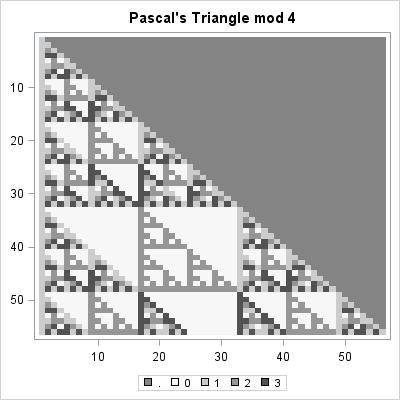The "mod 4" result is shown. The other heat maps are similar. Each shows a self-similar pattern. One of the surprising results of chaos theory and fractals is that these complicated self-similar structures can arise from simple iterative arithmetic operations (adding adjacent elements) followed by a modular operation.

### Creating larger triangles

The astute reader will have noticed that 56 rows is a curious number. Why not 64 rows? Or 100? The answer is that the modular operations require that the numbers in Pascal's triangle be exactly representable as an integer. Although you can compute more than 1,000 rows of Pascal's triangle in double precision, at some point the numbers grow so large that they can no longer be represented exactly with an 8-byte integer.

You can use the SAS CONSTANT function to find the largest integer, B, such that smaller integers (in magnitude) are exactly represented by an 8-byte numeric value. It turns out that the largest value in a Pascal triangle with k rows is "k choose floor(k/2)," and this value exceeds B when k=57, as shown by the following statements. Thus the modulo operations will become inaccurate for k>56.

```B = constant('ExactInt'); print B;   k = T(55:58); c = comb(k, floor(k/2)); print k c[L="k choose [k/2]"] (c<B)[L="Exact?"];```I think Pascal's triangle is very cool. When did you first encountered Pascal's triangle? Were you fascinated or bored? Share your story in the comments.

tags: Heat maps, Just for Fun, MathMy previous blog post describes how to implement Conway's Game of Life by using the dynamically linked graphics in SAS/IML Studio. But the Game of Life is not the only kind of cellular automata. This article describes a system of cellular automata that is known as Wolfram's Rule 30. In this blog post I show how to compute and visualize this system in SAS by using traditional PROC IML and a simple heat map.

### Wolfram's Rule 30

Conway's Game of Life is a set of rules for evolving cellular automata on a two-dimensional grid. However, one-dimensional automata are simpler to describe and to compute. A well-known one-dimensional example is Wolfram's Rule 30 (1983, Rev. Mod. Phys.). Consider a sequence of binary symbols, such as 0 and 1. An initial configuration determines the next generation by using the following two rules for each cell:

1. If the cell and its right-hand neighbor are both 0, then the new value of the cell is the value of its left-hand neighbor.
2. Otherwise, the new value of the cell is the opposite of the value of its left-hand neighbor.

The rules are often summarized by using three-term sequences that specify the value of the left, center, and right cells. The sequence 100 means that the center cell is 0, its left neighbor is 1, and its right neighbor is 0. According to the first rule, the value of the center cell for the next generation will be 1. The sequence 000 means that the center cell and both its neighbors are 0. According to the first rule, the value of the center cell for the next generation will be 0. All other sequences are governed by the second rule. For example, the sequence 010 implies that the center cell for the next generation will be 1, which is the opposite of the value of the left neighbor.

There are several ways that you can implement Rule 30 in a matrix language such as SAS/IML. The following SAS/IML program defines a function that returns the next generation of binary values when given the values for the current generation. As for the Game of Life, I define the leftmost and rightmost cells to be neighbors. The evolution function is vectorized, which means that no loops are used to compute a new generation from the previous generation. The second function computes each new generation as a row in a matrix. You can print that matrix or use the HEATDISC subroutine in SAS/IML 13.1 to create a black-and-white heat map of the result.

```proc iml; start Rule30Evolve(x); /* x is binary row vector */ y = x[ncol(X)] || x || x; /* extend x periodically */ N = ncol(y); j = 2:N-1; xj = y[,j]; /* the original cells */ R = y[,j+1]; L = y[,j-1]; /* R=right neighbor; L=left neighbor */ idxSame = loc(xj=0 & R=0); /* x00 --> center cell is x */ if ^IsEmpty(idxSame) then y[,idxSame+1] = L[,idxSame]; idxDiff = setdif(1:N-2, idxSame); /* otherwise, center cell is ^x */ if ^IsEmpty(idxDiff) then y[,idxDiff+1] = ^L[,idxDiff]; return( y[,j] ); finish;   start Rule30(x0, Iters=ceil(ncol(x0)/2)); N = ncol(x0); M = j(Iters,N,0); /* initialize array to 0 */ M[1,] = x0; /* set initial configuration */ do i = 2 to Iters; M[i,] = Rule30Evolve(M[i-1,]); /* put i_th generation into i_th row */ end; return( M ); finish;   N = 101; x0 = j(1,N,0); /* initialize array to 0 */ x0[ceil(N/2)] = 1; /* set initial condition for 1st row */ M = Rule30(x0, N); ods graphics / width=800px height=600px; call heatmapdisc(M) displayoutlines=0 colorramp={White Black} title = "Wolfram's Rule 30 (N=101)";```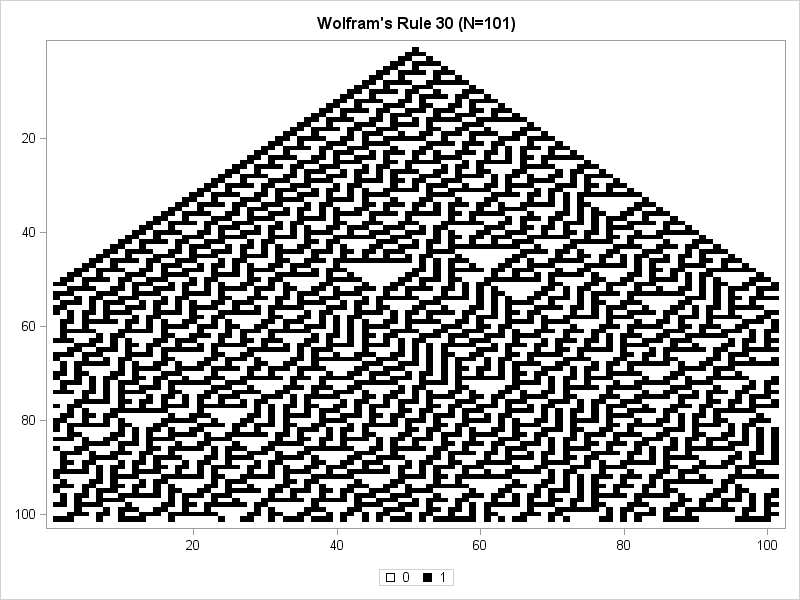The discrete heat map uses white to indicate cells that are 0 and black to indicate cells that are 1. The initial configuration has 100 cells of white and a single black cell in the middle of the array. That configuration is shown in the first row of the heat map. (Click to enlarge.) The second generation has three black cells in the middle, as shown in the second row. The central region of cells grows two cells larger for each generation. For the first 50 generations, the left boundary is always 001 and the right boundary alternates between 111 and 001. The pattern of the interior cells is not periodic, although certain interesting patterns appear, such as "martini glasses" of various sizes.

After 50 generations, the left and right edges begin to interact in a nontrivial way. Periodicity at the edges is replaced by irregular patterns.

Supposedly the evolution of the middle cell of the array generates nearly random 0-1 values. Mathematica, a computer software package created by Stephen Wolfram, uses the central column of a Rule-30 cellular automata as a random number generator. From a simple deterministic rule comes complex behavior that seems indistinguishable from randomness.

The heat map shows all generations at a single glance by stacking them as rows in a single image. This is different from the Game of Life visualization, which used animation to show how an initial configuration evolves from one generation to the next. You can also use animation to visualize the Rule-30 system. The following animated GIF shows the same 100 generations of an initial configuration that consists of a single black cell in the middle of a 101-cell array. This animation shows how that configuration evolves in time.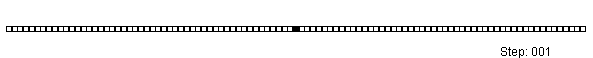It is fun to play with Rule 30. To begin, I suggest an array of 21 cells that evolves for 20 generations. The resulting black-and-white heat map is small enough to study, and you can use the DISPLAYOUTLINES=1 option to better visualize which cells are white during the evolution. You can invent your own initial configuration or use random initial conditions, as shown below:

```N = 21; x0 = T( randfun(N, "Bernoulli", 4/N) ); /* random; expect 4 black cells */ M = Rule30(x0); call heatmapdisc(M) displayoutlines=1 colorramp={White Black} title = "Wolfram's Rule 30 (N=21)";```

Enjoy!Have you ever looked as a statistical graph that uses bright garish colors and thought, "Why in the world did that guy choose those awful colors?" Don't be "that guy"! Your choice of colors for a graph can make a huge difference in how well your visualization is perceived by the reader. This is especially true for heat maps and choropleth maps in which the colors of small area elements must be distinguishable. This article describes how you can choose good color schemes for heat maps and choropleth maps. Most of the article is language-independent, but at the end I show how to create graphs with good color schemes in SAS.

### All color schemes are not created equal

I first became aware of the importance of color design in statistical graphics when I looked at the incredibly beautiful Atlas of United States Mortality by Linda Pickle and her colleagues (1997).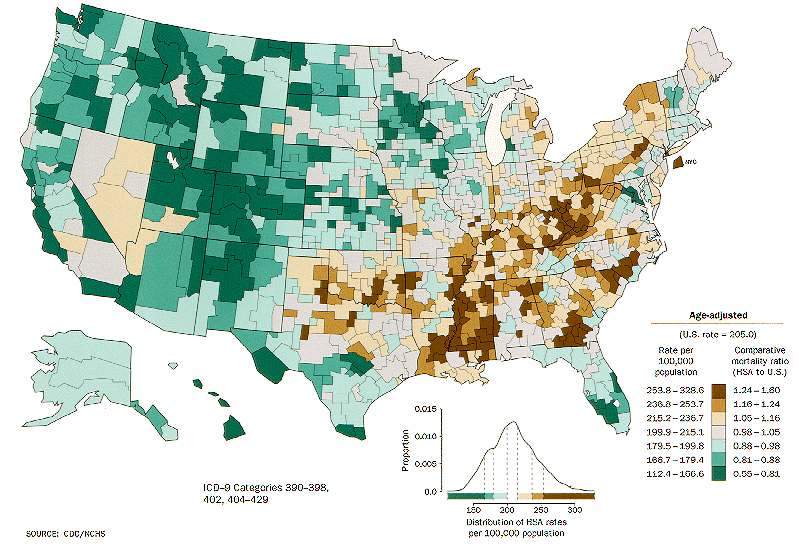One of the reasons that the Atlas is so successful at conveying complex statistical data on maps is that it uses carefully designed color schemes and visualization strategies that were the result of pioneering research by Cynthia Brewer, Dan Carr, and many others. An example from the Atlas is shown to the left. The color schemes were designed so that one color does not visually dominate the others. Bright reds and yellows are nowhere to be found. Fully saturated colors are avoided in favor of slightly muted colors. The result is that graphs in the Atlas enable you to see variations in the data without being biased by visual artifacts that can result from poor color choices.

Choosing good colors for maps and graphs is not easy, which is why every statistician and graphics designer should know about Cynthia Brewer's ColorBrewer.org web site. The web site enables you to choose from dozens of color schemes that were carefully chosen to be aesthetically pleasing as well as statistically unbiased.

### Sequential, diverging, and qualitative schemes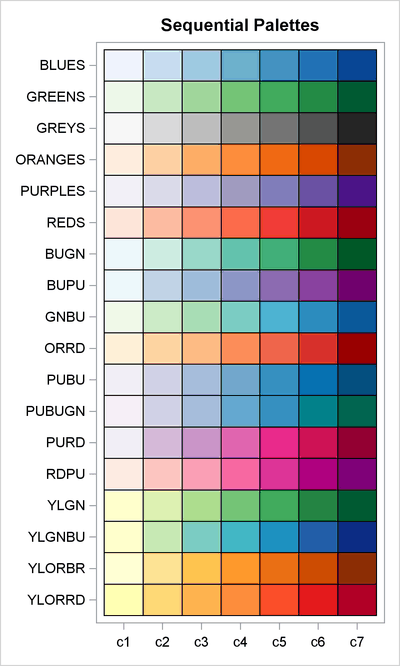Brewer's color schemes are implemented in SAS/IML 13.1 software through the PALETTE function. (Other statistical languages also provide access to these schemes, such as via the RColorBrewer package in R.) The PALETTE function enables you to get an array of colors by knowing the name of a color scheme. Some of the available color schemes are shown at the left. Brewer's "BLUES" color scheme is single-hue progression that is similar to the two-color ramp in the SAS HTMLBlue ODS style. The "YLORRD" color scheme is a yellow-orange-red sequential color ramp that shows a blended progression from yellow to red.

The color ramps at the left are appropriate for visualizing data when it is important to differentiate high values from low values. For these so-called sequential color schemes, the reference level is the lowest value.

There are other possible color schemes. When the reference value is in the middle of the data range (such as zero or an average value), you should use a diverging color scheme, which uses a neutral color for the reference value. Low values are represented by using one hue and high values by using a different hue.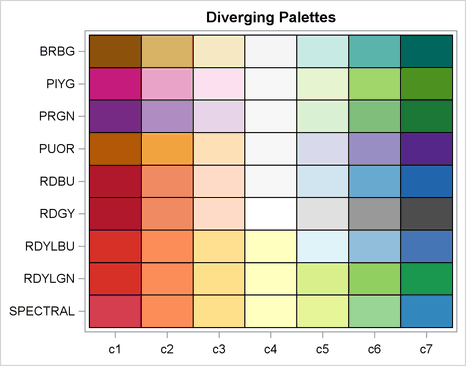Examples of diverging color schemes are shown to the right. The first diverging color scheme ("BRBG" for brown-blue-green) is the default color ramp that is used to construct heat maps of discrete data in SAS/IML software. For example, a five-color version of that color ramp was used to visualize a binned correlation matrix in my recent article about how to create heat maps with a discrete color ramp. The "BDBU" scheme (a red-blue bipolar progression) is often used when one extreme is "good" (blue) and the other extreme is "bad" (red). The "SPECTRAL" color scheme is a useful alternative to the often-seen "rainbow" color ramp, which is notoriously bad for visualization and should be avoided. A spectral progression is often used for weather-related intensities, such as storm severity.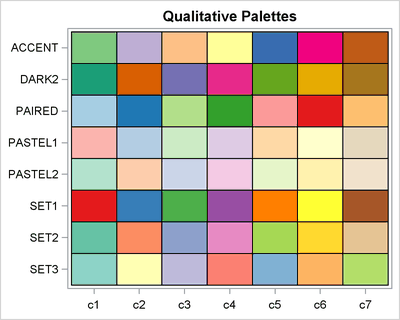Finally, there are qualitative color schemes, as shown to the left. These schemes are useful for visualizing data that have no inherent ordering, such as ethnicities of people, different diseases, types of music, and other nominal categories.

### Using color schemes with heat maps in SAS

Although these color schemes were designed for choropleth maps, the same principles are useful for designing heat maps with a small number of discrete categories. You can use the PALETTE function in SAS/IML software to choose effective colors for heat maps and other statistical graphics.

The PALETTE function is easy to use: Specify the name of the color scheme and the number of colors that you want to generate. These schemes are designed for a small number of discrete categories, so the schemes support between eight and 12 distinct and distinguishable colors.

As an example, suppose that you have computed a binned correlation matrix, but you want to override the default color scheme. For your application, you want negative values to be red and positive to be blue, so you decide on the bipolar "RDBU" color scheme. The following SAS/IML statements continue the example from my previous post:

```/* The disCorr matrix is defined in the previous example. */ ramp = palette("RDBU", 5); /* create diverging 5-color ramp */ call HeatmapDisc(disCorr) colorramp=ramp /* use COLORRAMP= option */ title="Binned Correlations" xvalues=varNames yvalues=varNames;```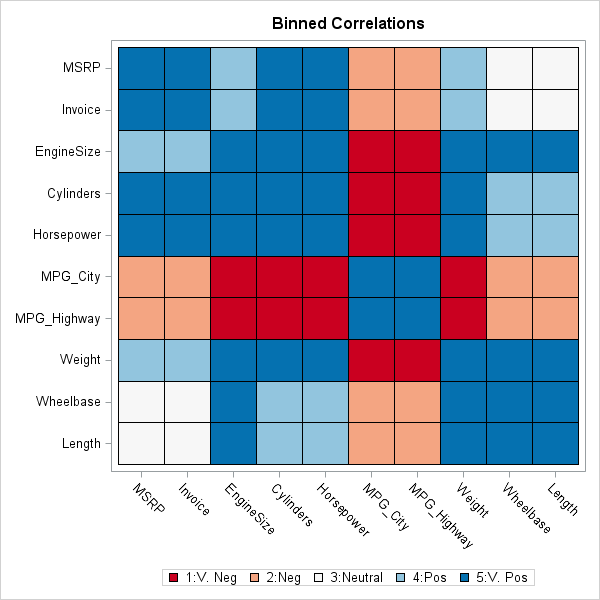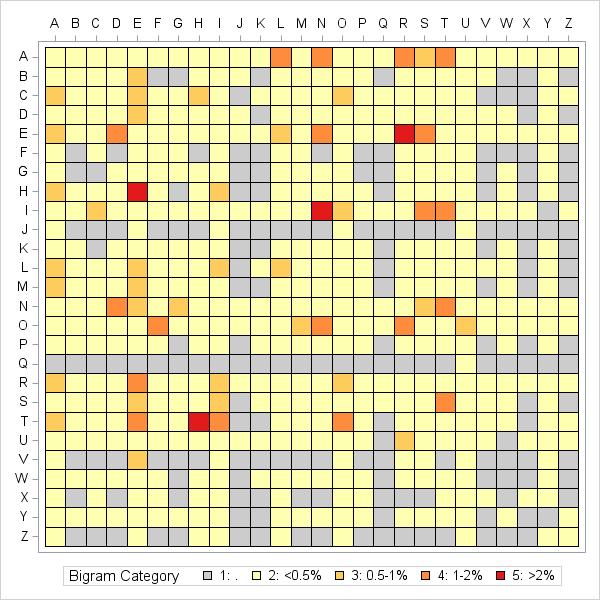As a second example, the heat map at the left use a discrete set of colors to visualize the most frequently appearing bigrams (two-letter combinations) in an English corpus. The values of the matrix have been binned into five categories. Red cells indicate bigrms that appear more than 2% of the time. Dark orange cells indicate bigrams that appear between 1% and 2%. Light orange represents between 0.5% and 1%; light yellow represents less than 0.5% of the time. Gray cells represent bigrams that appear rarely or not at all. You can download the SAS program that computes this discrete heat map of bigram frequency.

Incidentally, you can also use the PALETTE function as an easy way to generate a continuous color ramp. You can use the PALETTE function to obtain three or five anchor colors and interpolate between those colors to visualize intermediate values. In my article about hexagonal bin plots, the continuous color map used for the density map of ZIP codes was created by using the colors from the call palette("YLORRD",5).

What method do you use to choose colors for maps or heat maps that display categories? Do you have a favorite color scheme? Leave a comment.In a previous article I introduced the HEATMAPCONT subroutine in SAS/IML 13.1, which makes it easy to visualize matrices by using heat maps with continuous color ramps. This article introduces a companion subroutine. The HEATMAPDISC subroutine, which also requires SAS/IML 13.1, is designed to visualize matrices that have a small number of unique values. This includes zero-one matrices, design matrices, and matrices of binned data.

I have previously described how to use the Graph Template Language (GTL) in SAS to construct a heat map with a discrete color ramp. The HEATMAPDISC subroutine simplifies this process by providing an easy-to-use interface for creating a heat map of a SAS/IML matrix. The HEATMAPDISC routine supports a subset of the features that the GTL provides.

### A two-value matrix: The Hadamard design matrix

In my previous article about discrete heat maps, I used a Hadamard matrix as an example. The Hadamard matrix is used to make orthogonal array experimental designs. The following SAS/IML statement creates a 64 x 64 matrix that contains the values 1 and –1 and calls the HEATMAPDISC subroutine to visualize the matrix:

```proc iml; h = hadamard(64); /* 64 x 64 Hadamard matrix */ ods graphics / width=600px height=600px; /* make heat map square */ run HeatmapDisc(h);```The resulting heat map is shown to the left. (Click to enlarge.) You can see by inspection that the matrix is symmetric. Furthermore, with the exception of the first row and column, all rows and columns have an equal number of +1 and –1 values. There are also certain rows and columns (related to powers of 2) whose structure is extremely regular: a repeating sequence of +1s followed by a sequence of the same number of –1s. Clearly the heat map enables you to examine the patterns in the Hadamard matrix better than staring at a printed array of numbers.

### A binned correlation matrix

In my previous article about how to create a heat map, I used a correlation matrix as an example of a matrix that researchers might want to visualize by using a heat map with a continuous color ramp. However, sometimes the exact values in the matrix are unimportant. You might not care that one pair of variables has a correlation of 0.78 whereas another pair has a correlation of 0.82. Instead, you might want to know which variables are positively correlated, which are uncorrelated, and which are negatively correlated.

To accomplish this task, you can bin the correlations. Suppose that you decide to bin the correlations into the following five categories:

1. Very Negative: Correlations in the range [–1, –0.6).
2. Negative: Correlations in the range [–0.6, –0.2).
3. Neutral: Correlations in the range [–0.2, 0.2).
4. Postive: Correlations in the range [0.2, 0.6).
5. Very Postive: Correlations in the range [0.6, 1].

You can use the BIN function in SAS/IML to discover which elements of a correlation matrix belong to each category. You can then create a new matrix whose values are the categories. The following statements create a binned matrix from the correlations of 10 numerical variables in the Sashelp.Cars data set. The HEATMAPDISC subroutines creates a heat map of the result:

```use Sashelp.Cars; read all var _NUM_ into Y[c=varNames]; close; corr = corr(Y);   /* bin correlations into five categories */ Labl = {'1:V. Neg','2:Neg','3:Neutral','4:Pos','5:V. Pos'}; /* labels */ bins= bin(corr, {-1, -0.6, -0.2, 0.2, 0.6, 1}); /* BIN returns 1-5 */ disCorr = shape(Labl[bins], nrow(corr)); /* categorical matrix */ call HeatmapDisc(disCorr) title="Binned Correlations" xvalues=varNames yvalues=varNames;```The heat map of the binned correlations is shown to the left. The fuel economy variables are very negatively correlated (dark brown) with the variables that represent the size and power of the engine. The fuel economy variables are moderately negatively correlated (light brown) with the vehicle price and the physical size of the vehicles. The size of the vehicles and the price are practically uncorrelated (white). The light and dark shades of blue-green indicate pairs of vehicles that are moderately and strongly positively correlated.

The HEATMAPDISC subroutine is a powerful tool because it enables you to easily create a heat map for matrices that contain a small number of values. Do you have an application in mind for creating a heat map with a discrete color ramp? Let me know your story in the comments.

You might be wondering how the colors in the heat maps were chosen and whether you can control the colors in the color ramp. My next blog post will discuss choosing colors for a discrete color ramp.